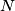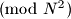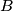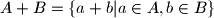### IMO Shortlist 1999 problem C4

Kvaliteta:
Avg: 3,5
Težina:
Avg: 7,0
Let$A$ be a set of$N$ residues$\pmod{N^{2}}$. Prove that there exists a set$B$ of$N$ residues$\pmod{N^{2}}$ such that$A + B = \{a+b|a \in A, b \in B\}$ contains at least half of all the residues$\pmod{N^{2}}$.
Izvor: Međunarodna matematička olimpijada, shortlist 1999